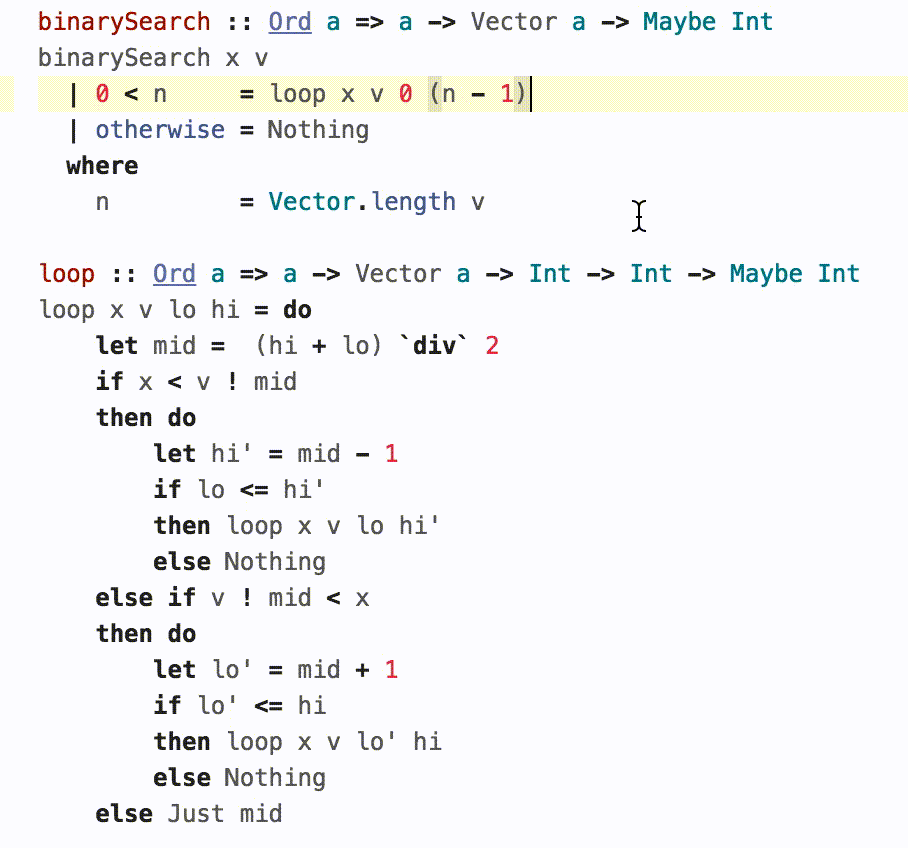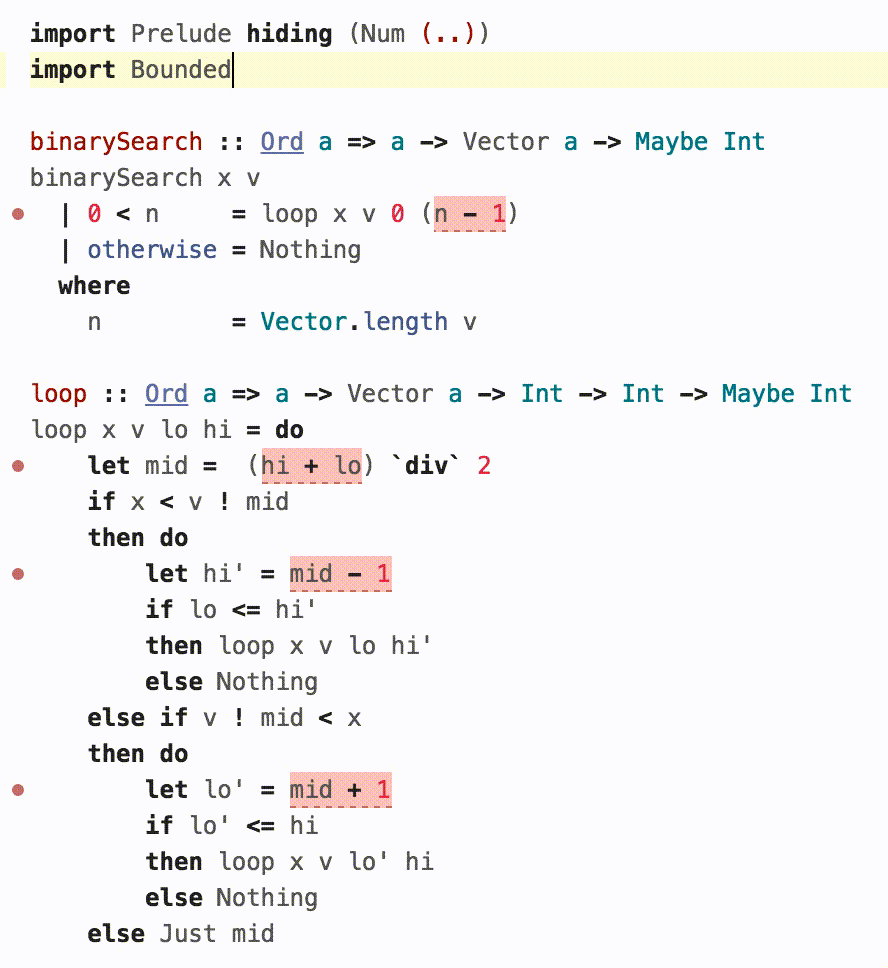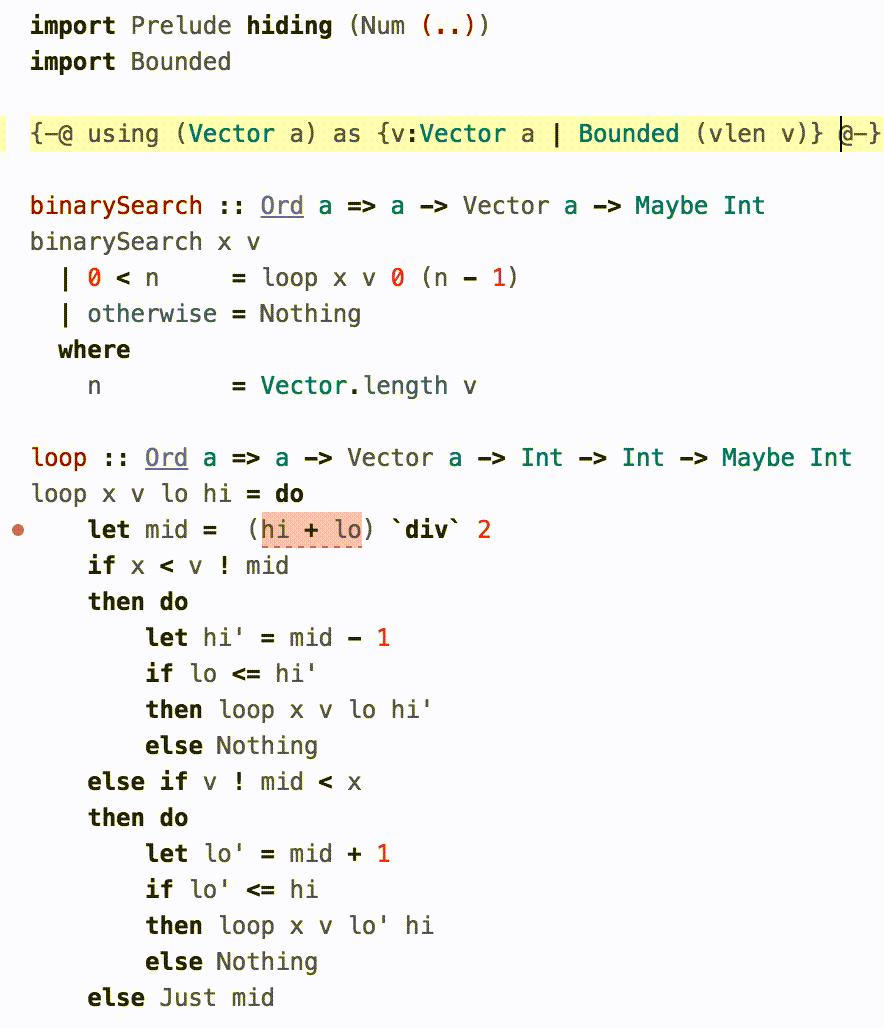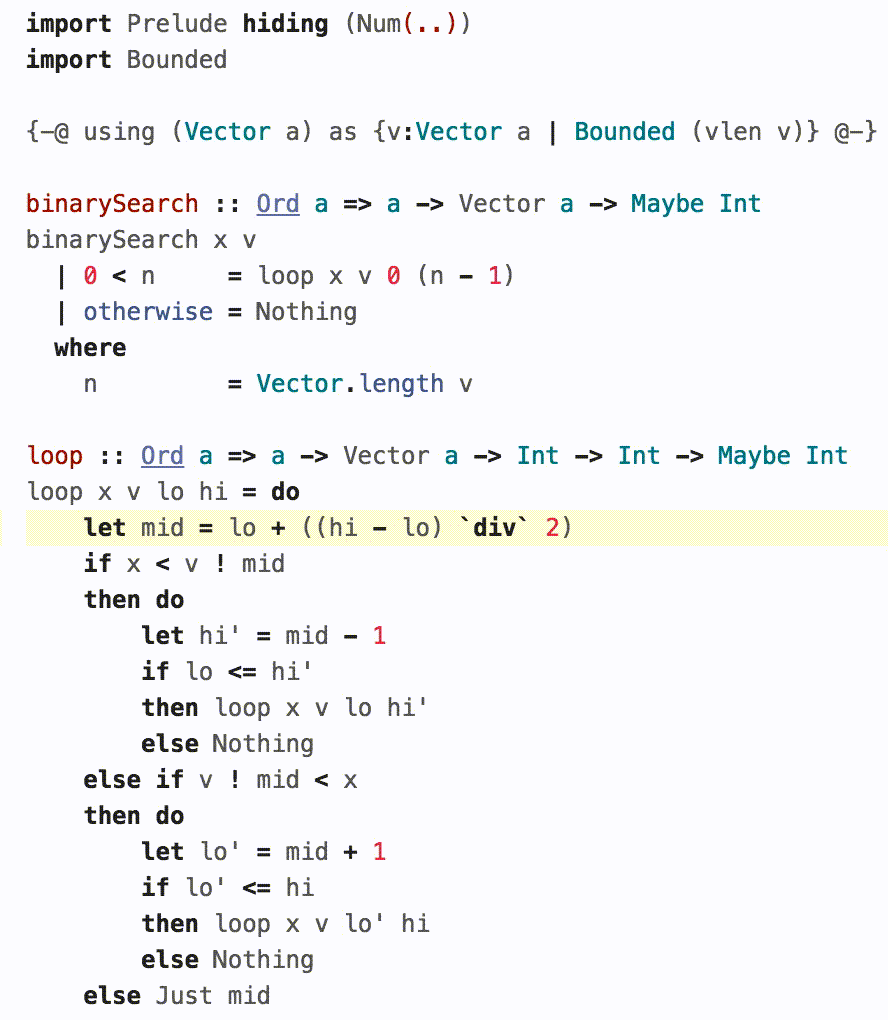# Arithmetic Overflows

Computers are great at crunching numbers. However, if programmers aren't careful, their machines can end up biting off more than they can chew: simple arithmetic operations over very large (or very tiny) inputs can overflow leading to bizarre crashes or vulnerabilities. For example, Joshua Bloch's classic post argues that nearly all binary searches are broken due to integer overflows. Lets see how we can teach LiquidHaskell to spot such overflows.

## 1. The Problem¶

LiquidHaskell, like some programmers, likes to make believe that Int represents the set of integers. For example, you might define a function plus as:

51: {-@ plus :: x:Int -> y:Int -> {v:Int | v == x + y} @-}
52: plus :: Int -> Int -> Int
53: x1:Int -> x2:Int -> {v : Int | v == x1 + x2}plus Intx Inty = {v : Int | v == y}x x1:Int -> x2:Int -> {v : Int | v == x1 + x2}Prelude.+ y


The output type of the function states that the returned value is equal to the \emph{logical} result of adding the two inputs.

The above signature lets us "prove" facts like addition by one yields a bigger number:

63: {-@ monoPlus :: Int -> {v:Bool | v <=> true } @-}
64: monoPlus :: Int -> Bool
65: Int -> {v : Bool | v <=> true}monoPlus Intx = {v : Int | v == x}x x1:Int -> x2:Int -> {v : Bool | v <=> x1 < x2}< {v : x1:Int -> x2:Int -> {v : Int | v == x1 + x2} | v == Bounded.plus}plus x 1


Unfortunately, the signature for plus and hence, the above "fact" are both lies.

LH checks plus as the same signature is assumed for the primitive Int addition operator Prelude.+. LH has to assume some signature for this "foreign" machine operation, and by default, LH assumes that machine addition behaves like logical addition.

However, this assumption, and its consequences are only true upto a point:

λ>  monoPlus 0
True
λ>  monoPlus 100
True
λ>  monoPlus 10000
True
λ>  monoPlus 1000000
True


Once we get to maxBound at the very edge of Int, a tiny bump is enough to send us tumbling backwards into a twilight zone.

λ> monoPlus maxBound
False

λ> plus maxBound 1
-9223372036854775808


## 2. Keeping Int In Their Place¶

The news isn't all bad: the glass half full view is that for "reasonable" values like 10, 100, 10000 and 1000000, the machine's arithmetic is the same as logical arithmetic. Lets see how to impart this wisdom to LH. We do this in two steps: define the biggest Int value, and then, use this value to type the arithmetic operations.

A. The Biggest Int

First, we need a way to talk about "the edge" -- i.e. the largest Int value at which overflows occur.

We could use the concrete number

λ> maxBound :: Int
9223372036854775807


However, instead of hardwiring a particular number, a more general strategy is to define a symbolic constant maxInt to represent any arbitrary overflow value and thus, make the type checking robust to different machine integer widths.

135: -- defines an Int constant called maxInt
136: {-@ measure maxInt :: Int @-}


To tell LH that maxInt is the "biggest" Int, we write a predicate that describes values bounded by maxInt:

144: {-@ predicate Bounded N = 0 < N + maxInt && N < maxInt @-}


Thus, Bounded n means that the number n is in the range [-maxInt, maxInt].

B. Bounded Machine Arithmetic

Next, we can assign the machine arithmetic operations types that properly capture the possibility of arithmetic overflows. Here are two possible specifications.

Strict: Thou Shalt Not Overflow A strict specification simply prohibits any overflow:

160: {-@ plusStrict :: x:Int -> y:{Int|Bounded(x+y)} -> {v:Int|v = x+y} @-}
161: x1:Int -> x2:{y : Int | 0 < (x1 + y) + maxInt
&& x1 + y < maxInt} -> {v : Int | v == x1 + x2}plusStrict Intx {y : Int | 0 < (x + y) + maxInt
&& x + y < maxInt}y = {v : Int | 0 < (x + v) + maxInt
&& x + v < maxInt
&& v == y}x x1:Int -> x2:Int -> {v : Int | v == x1 + x2}Prelude.+ y


The inputs x and y must be such that the result is Bounded, and in that case, the output value is indeed their logical sum.

Lazy: Overflow at Thine Own Risk Instead, a lazy specification could permit overflows but gives no guarantees about the output when they occur.

172: {-@ plusLazy :: x:Int -> y:Int -> {v:Int|Bounded(x+y) => v = x+y} @-}
173: x1:Int -> x2:Int -> {v : Int | 0 < (x1 + x2) + maxInt
&& x1 + x2 < maxInt => v == x1 + x2}plusLazy Intx Inty = {v : Int | v == y}x x1:Int -> x2:Int -> {v : Int | v == x1 + x2}Prelude.+ y


The lazy specification says that while plusLazy can be called with any values you like, the result is the logical sum only if there is no overflow.

To understand the difference between the two specifications, lets revisit the monoPlus property using the new machine-arithmetic sensitive signatures:

188: {-@ monoPlusStrict :: Int -> {v:Bool | v <=> true } @-}
189: Int -> {v : Bool | v <=> true}monoPlusStrict Intx = {v : Int | v == x}x x1:Int -> x2:Int -> {v : Bool | v <=> x1 < x2}< {v : x1:Int -> x2:{v : Int | 0 < (x1 + v) + maxInt
&& x1 + v < maxInt} -> {v : Int | v == x1 + x2} | v == Bounded.plusStrict}plusStrict x 1
190:
191: {-@ monoPlusLazy :: Int -> {v:Bool | v <=> true } @-}
192: Int -> {v : Bool | v <=> true}monoPlusLazy Intx = {v : Int | v == x}x x1:Int -> x2:Int -> {v : Bool | v <=> x1 < x2}< {v : x1:Int -> x2:Int -> {v : Int | 0 < (x1 + x2) + maxInt
&& x1 + x2 < maxInt => v == x1 + x2} | v == Bounded.plusLazy}plusLazy x 1


Both are rejected by LH, since, as we saw earlier, the functions do not always evaluate to True. However, in the strict version the error is at the possibly overflowing call to plusStrict. In the lazy version, the call to plusLazy is accepted, but as the returned value is some arbitrary Int (not the logical x+1), the comparison may return False hence the output is not always True.

Exercise: Can you fix the specification for monoPlusStrict and monoPlusLazy to get LH to verify the implementation?

## 3. A Typeclass for Machine Arithmetic¶

Its a bit inconvenient to write plusStrict and plusLazy, and really, we'd just like to write + and - and so on. We can do so, by tucking the above specifications into a bounded numeric typeclass whose signatures capture machine arithmetic. First, we define a BoundedNum variant of Num

220: class BoundedNum a where
221:   (+) :: a -> a -> a
222:   (-) :: a -> a -> a
223:   -- other operations ...


and now, we can define its Int instance just as wrappers around the Prelude operations:

230: instance BoundedNum Int where
231:   Intx x1:Int -> x2:{y : Int | 0 < (x1 + y) + maxInt
&& x1 + y < maxInt} -> {v : Int | v == x1 + x2}+ {y : Int | 0 < (x + y) + maxInt
&& x + y < maxInt}y = {v : Int | 0 < (x + v) + maxInt
&& x + v < maxInt
&& v == y}x x1:Int -> x2:Int -> {v : Int | v == x1 + x2}Prelude.+ y
232:   Intx x1:Int -> x2:{y : Int | 0 < (x1 - y) + maxInt
&& x1 - y < maxInt} -> {v : Int | v == x1 - x2}- {y : Int | 0 < (x - y) + maxInt
&& x - y < maxInt}y = {v : Int | 0 < (x - v) + maxInt
&& x - v < maxInt
&& v == y}x x1:Int -> x2:Int -> {v : Int | v == x1 - x2}Prelude.- y


Finally, we can tell LH that the above above instance obeys the (strict) specifications for machine arithmetic:

239: {-@ instance BoundedNum Int where
240:       + :: x:Int -> y:{Int | Bounded (x+y)} -> {v:Int | v == x+y };
241:       - :: x:Int -> y:{Int | Bounded (x-y)} -> {v:Int | v == x-y }
242:   @-}


With the above instance in scope, we can just use the plain + operator and have LH flag potential overflows:

249: {-@ mono :: Int -> {v:Bool | v <=> true} @-}
250: Int -> {v : Bool | v <=> true}mono Intx = {v : Int | v == x}x x1:Int -> x2:Int -> {v : Bool | v <=> x1 < x2}< Intx + 1


The above seems a bit paranoid. Do overflows really matter? And if they do, is it really practical to check for them using the above?

Joshua Bloch's famous article describes a tricky overflow bug in an implementation of binary-search that lay hidden in plain sight in classic textbooks and his own implementation in the JDK for nearly a decade. Gabriel Gonzalez wrote a lovely introduction to LH using binary-search as an example, and a careful reader pointed out that it had the same overflow bug!

Lets see how we might spot and fix such bugs using BoundedNum. (Hover over the images to animate.)

**A. Off by One** Lets begin by just using the default Num Int which ignores overflow. As Gabriel explains, LH flags a bunch of errors if we start the search with loop x v 0 n as the resulting search can access v at any index between 0 and n inclusive, which may lead to an out of bounds at n. We can fix the off-by-one by correcting the upper bound to n-1, at which point LH reports the code free of errors.**B. Lots of Overflows** To spot arithmetic overflows, we need only hide the default Prelude and instead import the BoundedNum instance described above. Upon doing so, LH flags a whole bunch of potential errors -- essentially *all* the arithmetic operations which seems rather dire!
**C. Vector Sizes are Bounded** Of course, things aren't _so_ bad. LH is missing the information that the size of any Vector must be Bounded. Once we inform LH about this invariant with the [using directive][lh-invariants], it infers that as the lo and hi indices are upper-bounded by the Vector's size, all the arithmetic on them is also Bounded and hence, free of overflows.**D. Staying In The Middle** Well, *almost* all. The one pesky pink highlight that remains is exactly the bug that Bloch made famous. Namely: the addition used to compute the new midpoint between lo and hi could overflow e.g. if the array was large and both those indices were near the end. To ensure the machine doesn't choke, we follow Bloch's suggestion and re-jigger the computation to instead compute the midpoint by splitting the difference between hi and lo! the code is now free of arithmetic overflows and truly memory safe.
Ranjit Jhala
2017-03-20